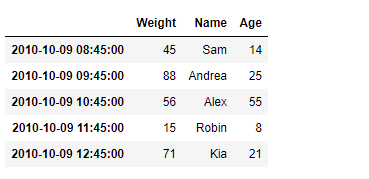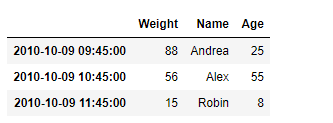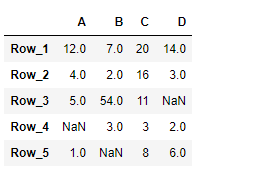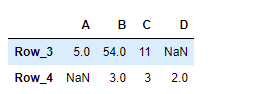# Python | Pandas DataFrame.truncate

• Difficulty Level : Easy
• Last Updated : 21 Feb, 2019

Pandas DataFrame is a two-dimensional size-mutable, potentially heterogeneous tabular data structure with labeled axes (rows and columns). Arithmetic operations align on both row and column labels. It can be thought of as a dict-like container for Series objects. This is the primary data structure of the Pandas.

Pandas` DataFrame.truncate()` function is used to truncate a Series or DataFrame before and after some index value. This is a useful shorthand for boolean indexing based on index values above or below certain thresholds.

Syntax: DataFrame.truncate(before=None, after=None, axis=None, copy=True)

Parameter :
before : Truncate all rows before this index value.
after : Truncate all rows after this index value.
axis : Axis to truncate. Truncates the index (rows) by default.
copy : Return a copy of the truncated section.

Returns : The truncated Series or DataFrame.

Example #1: Use `DataFrame.truncate()` function to truncate some entries before and after the passed labels of the given dataframe.

 `# importing pandas as pd ` `import` `pandas as pd ` ` `  `# Creating the DataFrame ` `df ``=` `pd.DataFrame({``'Weight'``:[``45``, ``88``, ``56``, ``15``, ``71``], ` `                   ``'Name'``:[``'Sam'``, ``'Andrea'``, ``'Alex'``, ``'Robin'``, ``'Kia'``], ` `                   ``'Age'``:[``14``, ``25``, ``55``, ``8``, ``21``]}) ` ` `  `# Create the index ` `index_ ``=` `pd.date_range(``'2010-10-09 08:45'``, periods ``=` `5``, freq ``=``'H'``) ` ` `  `# Set the index ` `df.index ``=` `index_ ` ` `  `# Print the DataFrame ` `print``(df) `

Output :Now we will use `DataFrame.truncate()` function to truncate the entries before ‘2010-10-09 09:45:00’ and after ‘2010-10-09 11:45:00’ in the given dataframe.

 `# return the truncated dataframe ` `result ``=` `df.truncate(before ``=` `'2010-10-09 09:45:00'``, after ``=` `'2010-10-09 11:45:00'``) ` ` `  `# Print the result ` `print``(result) `

Output :As we can see in the output, the `DataFrame.truncate()` function has successfully truncated the entries before and after the passed labels in the given dataframe.

Example #2: Use `DataFrame.truncate()` function to truncate some entries before and after the passed labels of the given dataframe.

 `# importing pandas as pd ` `import` `pandas as pd ` ` `  `# Creating the DataFrame ` `df ``=` `pd.DataFrame({``"A"``:[``12``, ``4``, ``5``, ``None``, ``1``],  ` `                   ``"B"``:[``7``, ``2``, ``54``, ``3``, ``None``],  ` `                   ``"C"``:[``20``, ``16``, ``11``, ``3``, ``8``],  ` `                   ``"D"``:[``14``, ``3``, ``None``, ``2``, ``6``]})  ` ` `  `# Create the index ` `index_ ``=` `[``'Row_1'``, ``'Row_2'``, ``'Row_3'``, ``'Row_4'``, ``'Row_5'``] ` ` `  `# Set the index ` `df.index ``=` `index_ ` ` `  `# Print the DataFrame ` `print``(df) `

Output :Now we will use `DataFrame.truncate()` function to truncate the entries before ‘Row_3’ and after ‘Row_4’ in the given dataframe.

 `# return the truncated dataframe ` `result ``=` `df.truncate(before ``=` `'Row_3'``, after ``=` `'Row_4'``) ` ` `  `# Print the result ` `print``(result) `

Output :As we can see in the output, the `DataFrame.truncate()` function has successfully truncated the entries before and after the passed labels in the given dataframe.

My Personal Notes arrow_drop_up
Recommended Articles
Page :